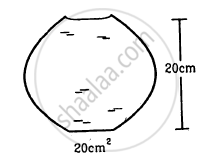Advertisement Remove all ads

# A Glass Full of Water Has a Bottom of Area 20 Cm2, Top of Area 20 Cm2, Height 20 Cm and Volume Half a Litre - Physics

Answer in Brief

A glass full of water has a bottom of area 20 cm2, top of area 20 cm2, height 20 cm and volume half a litre.
(a) Find the force exerted by the water on the bottom.
(b) Considering the equilibrium of the water, find the resultant force exerted by the sides of the glass on the water. Atmospheric pressure = 1.0 × 105 N/m2. Density of water 1000 kg/m3 and g = 10 m/s2. Take all numbers
to be exact.Advertisement Remove all ads

#### Solution

Given:

Atmospheric pressure, pa = 1.0 ×105N/m

Density of water, ρw =103kg/m3

Acceleration due to gravity, g =10m/s2

Volume of water , V = 500 mL ≈ 500g ≈ 0.5 kg

Area of the top of the glass, A = 20 m2
Height of the glass, h = 20 cm​

(a) Force exerted on the bottom of the glass = Atmospheric force + Force due to cylindrical water column or glass

=pa × A + A × h × ρw × g

=A(ρa + hρwg)

=20×10-4(105+20 ×10-2 ×103 ×10)

=204 N

(b) Let F​s be the force exerted by the sides of the glass. Now, from the free body diagram of water inside the glass, we can find out the resultant force exerted by the sides of the glass.

Thus, we have:

Pa × A + mg = A × h × ρw × g + Fs + Pa × A

⇒ mg = A × h × ρw × g + Fs

⇒ 0.5 × 1 = 20 × 10-4 × 20 × 10-2 × 10-3 × 10 + Fs

⇒ Fs = 5 - 4 = 1N (upward)

Is there an error in this question or solution?
Advertisement Remove all ads

#### APPEARS IN

HC Verma Class 11, 12 Concepts of Physics 1
Chapter 13 Fluid Mechanics
Q 4 | Page 273
Advertisement Remove all ads

#### Video TutorialsVIEW ALL 

Advertisement Remove all ads
Share
Notifications

View all notifications

Forgot password?